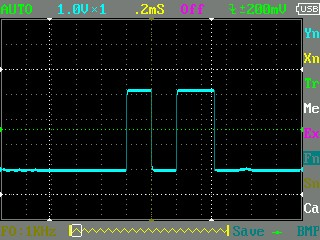Simple Pin control issue

• hi,
I just want to control an K thermometer with pin P4 (CS= chip select) and get data with P10 & 14 , so I wrote for my SipY on v3 expansion board :

import pycom
import machine
from machine import SPI
pycom.heartbeat(False)
print(machine.unique_id())
# configure the SPI master @ 9600
# this uses the SPI default pins for CLK, MISO (``P10``, ``P14``)

spi = SPI(0, mode=SPI.MASTER, baudrate=9600, polarity=0, phase=1, bits=12, firstbit=SPI.MSB)
# penser plus tard à mesurer D2
# initialiser CS sur P4 à 1
from machine import Pin
cs = Pin('P4', mode = Pin.OUT)
cs.value(1)

and I get at "run" an error after the machine Id :

b'\$\n\xc4\x00V\x86'
Traceback (most recent call last):
File "<stdin>", line 14, in <module>
ValueError: invalid argument(s) value
and line 14 is just : cs = Pin('P4', mode = Pin.OUT)

I do not understand , I just copied & adapted the documentation :
https://docs.pycom.io/chapter/firmwareapi/pycom/machine/Pin.html

where is my mistake please in Pin control ?
William

• Solution is here https://forum.pycom.io/post/21808 (bug in doc / Pin maps)

• @prx the frequency is given in Hz. Ans it does not matter whether the voltage is 3.2 or 3.3. The switch point is at about 1.6V. Vcc should be 3.3V. I had a max5595 running fine wirh your code.

• This post is deleted!

• @robert-hh
Thanks, yes P4 (CS) is the right pin, otherwise I would not have the reply I put you the signal as screen copy; I test also with read(2) : same result : 0 , I am not sure of a/ 10000 = 10 Mkz or 1 Mkz ? and b/threshold of the signal on Miso : I have this evening a max voltage at 3.2V : is the signal considered at "1" with 3.2V on SipY Miso pin? -
The same installation with 3.3V in Vcc was working on Arduino last week and gave me temperature's answer quickly.
best regards
William

• @prx You should use: spi.read(2), but still the value should be > 0
Edit: P4 is the 5th Pin from the edge. The first one is reset, not P0. I use to stumble about that.

• hi @robert-hh ,
I get 0 as temp , and data, event in 16 or 8 bits (SPI)
I write the following code :

import time
import pycom
import machine
import ubinascii
from machine import SPI, Pin
pycom.heartbeat(False)
print(ubinascii.hexlify(machine.unique_id()))
# this uses the SPI default pins for CLK, MISO (``P10``, ``P14``)
spi = SPI(0, mode=SPI.MASTER, baudrate=10000, polarity=0, phase=1, bits=16, firstbit=SPI.MSB)
# penser plus tard à mesurer D2

# initialiser CS on P4 to 1
from machine import Pin
cs = Pin('P4', mode = Pin.OUT)
cs.value(1)

def run():
time.sleep(.0500)
cs.value(0)
#time.sleep(.500)
cs.value(1)
#value = (data * 256 + data) >> 4
#print("Temperature: ", value/4)
print(data)

while True:
run()

but on P14 i get an answer signal see picture (DSO) !![0_1534748324300_IMG_000.BMP](Uploading 100%) Vmax =3.16V : so Miso port do not read it.
My Pin connexion on Max6675 is :

• Vcc= 3V3 (3ed pin on the top right)
• gnd
• SCK on P10
• CS on P4
• SO on P14 (Miso)
thank you by advance
Wiliam

• Thank you @robert-hh , I passed the "line 12" problem and will work on the following and post the result - yes it is a Max6675 for the moment. Thank you.

• @prx With my MAX6675 here, i extended your script to prin values:

import pycom
import machine
import ubinascii
from machine import SPI, Pin
pycom.heartbeat(False)
print(ubinascii.hexlify(machine.unique_id()))
# configure the SPI master @ 9600
# this uses the SPI default pins for CLK, MISO (``P10``, ``P14``)

spi = SPI(0, mode=SPI.MASTER, baudrate=10000, polarity=0, phase=1, bits=8, firstbit=SPI.MSB)
# penser plus tard à mesurer D2
# initialiser CS sur P4 à 1
cs = Pin('P4', mode = Pin.OUT)
cs.value(1)

def run():

cs.value(0)
cs.value(1)
value = (data * 256 + data) >> 4
print("Temperature: ", value/4)

run()

• @prx Just saw something else: the parameter bits could be wrong. from the documentation:
"bits is the width of each transfer, accepted values are 8, 16 and 32."
Error messages are sometimes off by one line.
If the device you try to talk to is a max6675, then the data size is 16 bits. If the device is just sending 12 bits, read 16 and ignore 4.
And about the data rate: according to the data sheet the max6675 can be clocked up to 5 MHz. So no need to use 9600.
Edit: 5 MHz do not work (skips a bit), but 1 MHz do,

• This post is deleted!